# The Hydrogen AtomSpherical polar coordinates The solution of the Schrodinger equation for the hydrogen atom is a formidable mathematical problem, but is of such fundamental importance that it will be treated in outline here. The solution is managed by separating the variables so that the wavefunction is represented by the product: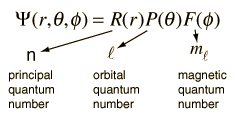The separation leads to three equations for the three spatial variables, and their solutions give rise to three quantum numbers associated with the hydrogen energy levels.
Index

Schrodinger equation concepts

Hydrogen concepts

 HyperPhysics***** Quantum Physics R Nave
Go Back

# Hydrogen Schrodinger Equation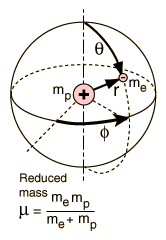The electron in the hydrogen atom sees a spherically symmetric potential, so it is logical to use spherical polar coordinates to develop the Schrodinger equation. The potential energy is simply that of a point charge: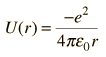The expanded form of the Schrodinger equation is shown below. Solving it involves separating the variables into the formThe starting point is the form of the Schrodinger equation: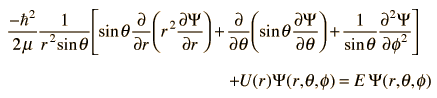Index

Schrodinger equation concepts

 HyperPhysics***** Quantum Physics R Nave
Go Back

# Quantum Numbers from Hydrogen Equations

The hydrogen atom solution requires finding solutions to the separated equations which obey the constraints on the wavefunction. The solution to the radial equation can exist only when a constant which arises in the solution is restricted to integer values. This gives the principal quantum number:Similarly, a constant arises in the colatitude equation which gives the orbital quantum number:Finally, constraints on the azimuthal equation give what is called the magnetic quantum number: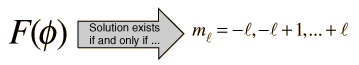### Discussion of concepts

Index

Schrodinger equation concepts

Hydrogen concepts

 HyperPhysics***** Quantum Physics R Nave
Go Back

# Separating the Hydrogen Equation

One of the approaches to solving a partial differential equation is to separate it into individual equations for each variable involved. The hydrogen Schrodinger equation is separable, and collecting all the radius-dependent terms and setting them equal to a constant gives the radial equation: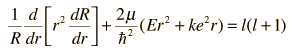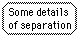Then the angular parts of the equation can be separated into a colatitude equation: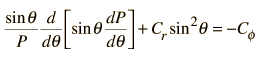and an azimuthal equation.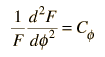Index

Schrodinger equation concepts

Hydrogen concepts

 HyperPhysics***** Quantum Physics R Nave
Go Back